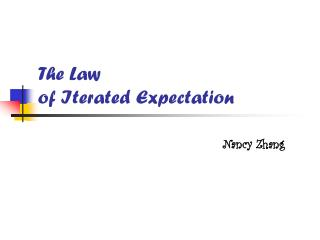DownloadDownload PresentationThe Law of I terated E xpectation

# The Law of I terated E xpectation

Download Presentation## The Law of I terated E xpectation

- - - - - - - - - - - - - - - - - - - - - - - - - - - E N D - - - - - - - - - - - - - - - - - - - - - - - - - - -
##### Presentation Transcript

1. The Law of Iterated Expectation Nancy Zhang

2. Easy Example 1bivariate random variables Let X=schooling of the person Y=monthly income of the person Solve the expectation of Y from the following table x=1 have an university degree or above x=2 don’t have an university degree

3. Example 1 (continued) Solution: E(X)=E(Y|X=1)*P(X=1) +E(Y|X=2)*P(X=2) • X=x1,x2…,xn • E (Y)=E(Y|X=x1)*P(X=x1)+E(Y|X=x2)*P(X=x2) +…+E(Y|X=xn)*P(Y|X=xn) =∑x E(Y|X=xi)*P (X=xi)

4. Example 1 (continued) Think E(Y|X=xi) as one random variable E(Y|X=xi) =Ai: E(Y)=∑x Ai*P(X=xi)=E(Ai)=E[E(Y|X=xi)]=E[μY|X] *This process is called average out X. Recall the formula: E(Y)= ∑x E(Y|X=xi)*P (X=xi) = E[E(Y|X=xi)] = E[μY|X]

5. Intermediate E(Y)= ∑x E(Y|X=xi)*P (X=xi) = E[E(Y|X=xi)] = E[μY|X] Example 2 trivariate random variables Let X=schooling of the person Y=monthly income of the person Z=gender (Z=1 men; Z=2 women) a. Check if there is salary discrimination on women b. Solve the expectation of Y from the following table

6. E(Y)= ∑x E(Y|X=xi)*P (X=xi) = E[E(Y|X=xi)] = E[μY|X] Example 2 (continued) Solution: Step 1:average out X a. When Z=1, Use the formula above, average out X: let: E(Y|X=xi,Z=1)=a1 E(A|Z=1)= ∑x E(Y|X=xi,Z=1)*P(X=xi) = E[E(Y|X=xi,Z=1)|Z=1] = 1200*0.5+400*0.5=800

7. E(Y)= ∑x E(Y|X=xi)*P (X=xi) = E[E(Y|X=xi)] = E[μY|X] Example 2 (continued) Similarly, when Z=2, average out X: E(A|Z=2) = ∑x E(Y|X=xi,Z=2)*P(X=xi) = 800*0.5+500*0.5=650 As 650<800, the conditional expectations suggest that there is salary discrimination on women.* *Note: self-created data, not based on real survey.

8. E(Y)= ∑x E(Y|X=xi)*P (X=xi) = E[E(Y|X=xi)] = E[μY|X] Example 2 (continued) Step 2: average out Z: Again use the formula for bivariate random numbers: E(Y)= ∑z E(A|Z=zi)*P(Z=zi) = E[E(A|Z=zi)] = E{E[E(Y|X, Z)|Z]} = 0.5*800+650*0.5=725

9. Hard The law of iterated expectation • For a bivariate random variables, X and Y: • E(Y) = E[E(Y|X)] • For a trivariate random variables, X, Y and Z • E(Y|X,Z) = conditional mean given X and Z. • E(Y|Z) = E[E(Y|X,Z)|Z] • E(Y|X) = E[E(Y|X,Z)|X] • E(Y) = E{E[E(Y|X,Z)|Z]} • (note: taking expectations in several iterations.)